[top.htm]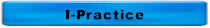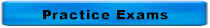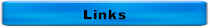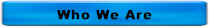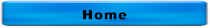Answers to More Collection Questions Click here to return to More Collection Questions Which of the following terms refers to groundwater seeping into a sanitary sewer? Exfiltration Filtration Infiltration Inflow The correct answer is C.  Infiltration   Hydrogen sulfide gas is most likely found in a collection system with a low pH or high pH?  The correct answer is Low pH   Chlorine ton cylinders at room temperature will deliver gas at a maximum rate of       150 lb cylinders        Ton Containers A.  10 pounds per day      100 pounds per day B.  25 pounds per day      250 pounds per day C.  40 pounds per day      450 pounds per day D.  150 pounds per day    2000 pounds per day The correct answer is C   Which of the following valves only permits flow to move in one direction? A.  Butterfly B.  Check C.  Gate D.  Plug The correct answer is B   The specific gravity of a colloidal solid is very close to 1.  This means that the colloid A.  Will float within an hour B.  Will settle within an hour C.  Won't settle or float The correct answer is C   The specific gravity of settleable solid is A.  1 B.  Greater than 1 C.  Less than 1 The correct answer is B   The specific gravity of a floatable solid is A.  1 B.  Greater than 1 C.  Less than 1 The correct answer is C   The specific gravity of chlorine gas is A.  1 B.  Greater than 1 C.  Less than 1 The correct answer is B   Which of the following is correct? A.  A is the follower and B is the lantern ring B.  A is the lantern ring and B is a packing ring C.  A is a packing ring and B is the lantern ring D.  A is a packing ring and B is the follower The correct answer is C.  A is a packing ring and B is the lantern ring   pH measures A.  H+ B.  OH- C.  O2 D.  Phosphorus The correct answer is A.  H+  -- pH measures the hydrogen ion concentration  How do you know that your pump is cavitating? A.  It doesn't sound right B.  It runs hot C.  It won't start D.   All of the above The correct answer is A.  It doesn't sound right. A pump that's cavitating sounds like it's pumps rocks or gravel.   An atom is composed of A.  Electrons B.  Neutrons C.  Protons D.  All of the above The correct answer is D.  All of the Above   Throttling the discharge valve on a centrifugal pump will the likely ______ temperature of the motor and ______ the temperature of the pump A.  Increase, increase B.  Increase, reduce C.  Reduce, increase D.  Reduce, reduce The correct answer is C.  Reduce, increase A, B, and D are incorrect because throttling the discharge valve on a centrifugal pump will the likely reduce temperature of the motor and increase the temperature of the pump  The chemical symbol for chlorine gas is A.  Cl B.  Cl- C.  Cl2 D.  HOCl The correct answer is C.  Cl2 A is incorrect because this is chemical symbol for chlorine not chlorine gas B is incorrect because this is the chemical symbol for chloride D is incorrect because this is the chemical formula for hypochlorite  Which of the following is the indicator used for pathogens? A.  Amoebic dysentery B.  E. coli C.  Salmonella typhosa D.  V. comma The correct answer is B.  E. coli A is incorrect because this is a pathogen, not an indicator C is incorrect because this is a pathogen (cholera), not an indicator D is incorrect because this is a pathogen (typhoid), not an indicator  Pump suction and discharge pressures are usually measured in A.  gpm B.  kWh   C.  mg/L D.  psi The correct answer is D.  psi A is incorrect because this is a measure of flow B is incorrect because this is a measure of energy used C is incorrect because this is a measure of concentration   The PE for flow is ____ gpcd and PE for BOD is _____ lbs BOD/person/day. A.  0.17 for both B.  0.17 and 100 C.  100 and 0.17 D.  100 for both The correct answer is C.  100 and 0.17 B, C, D are incorrect because the PE (population equivalent) for flow is 100 gpcd (gallons per capita per day and 0.17 lbs BOD/person/day   1.  D 2.  A   What is the minimum recommended velocity in sanitary sewers? A.  0 fps B.  1 fps C.  2 fps D.  10 fps The correct answer is C.  2 fps C is the minimum recommended velocity in non-aerated grit chambers D is the maximum recommended velocity in sanitary sewers  For questions or comments, call 515-313-1159 Home